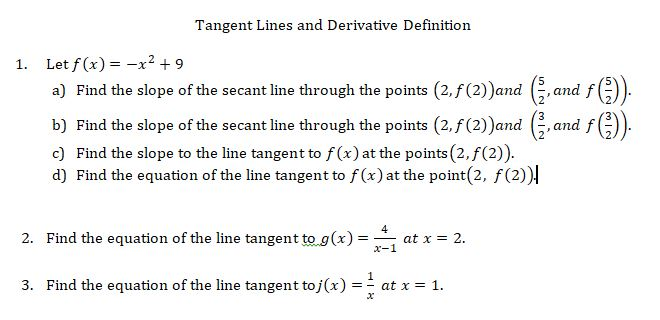# Tangent Lines and Derivative DefinitionLet f(x) x2 9a) Find the slope of the secant line through the points (2,f(2))and (,an1.b) Find the slope of the secant line through the points (2, f(2))and (G,andc) Find the slope to the line tangent to f(x) at the points (2, f(2)).d) Find the equation of the line tangent to f (x) at the point(2, f(2))4at x 2x-12. Find the equation of the line tangent to g(x)=3. Find the equation of the line tangent to j(x)at x 1х

Question
16 viewshelp_outlineImage TranscriptioncloseTangent Lines and Derivative Definition Let f(x) x2 9 a) Find the slope of the secant line through the points (2,f(2))and (,an 1. b) Find the slope of the secant line through the points (2, f(2))and (G,and c) Find the slope to the line tangent to f(x) at the points (2, f(2)). d) Find the equation of the line tangent to f (x) at the point(2, f(2)) 4 at x 2 x-1 2. Find the equation of the line tangent to g(x)= 3. Find the equation of the line tangent to j(x) at x 1 х fullscreen
check_circle

Step 1

We’ll answer the first question with three sub-parts since the exact one wasn’t specified. Please submit a new question specifying the one you’d like answered.

Step 2

Given,

Step 3

Slope of secant line...

### Want to see the full answer?

See Solution

#### Want to see this answer and more?

Solutions are written by subject experts who are available 24/7. Questions are typically answered within 1 hour.*

See Solution
*Response times may vary by subject and question.
Tagged in

### Derivative